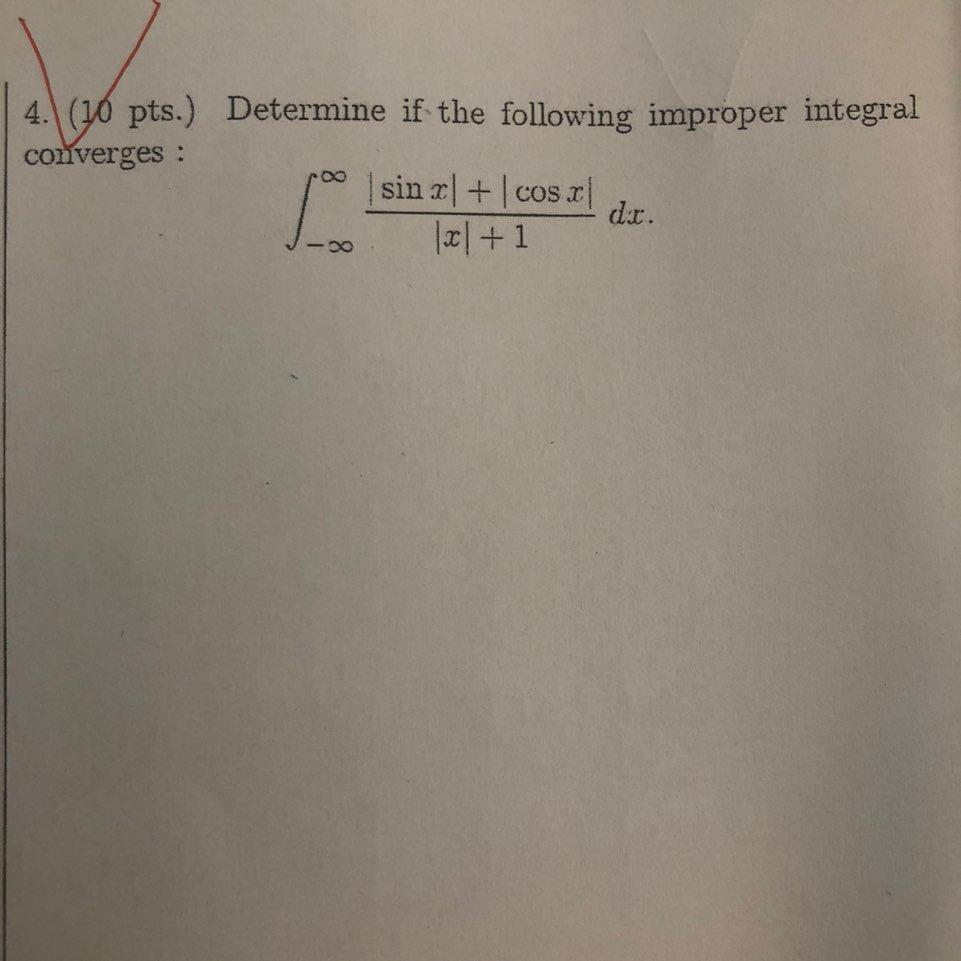### Create an Account

Home / Questions / 4. (10 pts.) Determine if the following improper integral converges : sin r| + cosr dir. al+1

# 4. (10 pts.) Determine if the following improper integral converges : sin r| + cosr dir. al+1

4. (10 pts.) Determine if the following improper integral converges : sin r| + cosr dir. al+1Apr 20 2021 View more View LessSubscribe To Get Solution